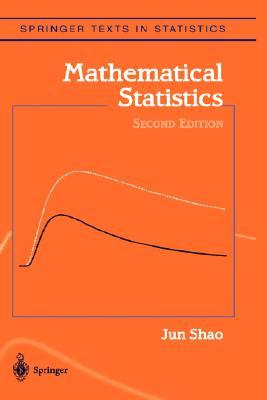## max vs. minAnother intriguing question on X validated (about an exercise in Jun Shao’s book) that made me realise a basic fact about exponential distributions. When considering two Exponential random variables X and Y with possibly different parameters λ and μ,  Z⁺=max{X,Y} is dependent on the event X>Y while Z⁻=min{X,Y} is not (and distributed as an Exponential variate with parameter λ+μ.) Furthermore, Z⁺ is distributed from a signed mixture$\frac{\lambda+\mu}{\mu}\mathcal Exp(\lambda)-\frac{\lambda}{\mu}\mathcal Exp(\lambda+\mu)$

conditionally on the event X>Y, meaning that there is no sufficient statistic of fixed dimension when given a sample of n realisations of Z⁺’s along with the indicators of the events X>Y…. This may explain why there exists an unbiased estimator of λ⁻¹-μ⁻¹ in this case and (apparently) not when replacing Z⁺ by Z⁻. (Even though the exercise asks for the UMVUE.)

This site uses Akismet to reduce spam. Learn how your comment data is processed.